# 一种简单而智能的方法：Python也能进行面部识别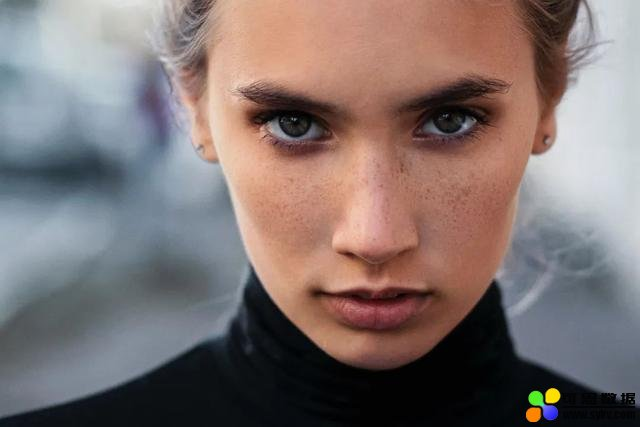Haar库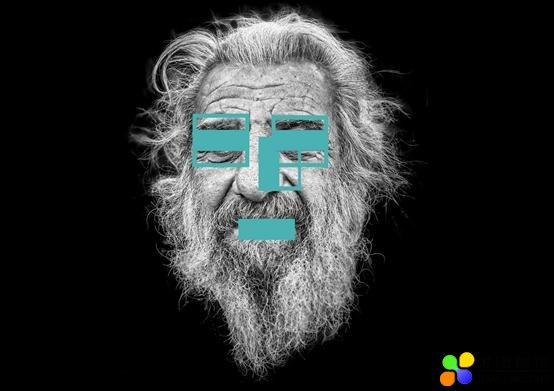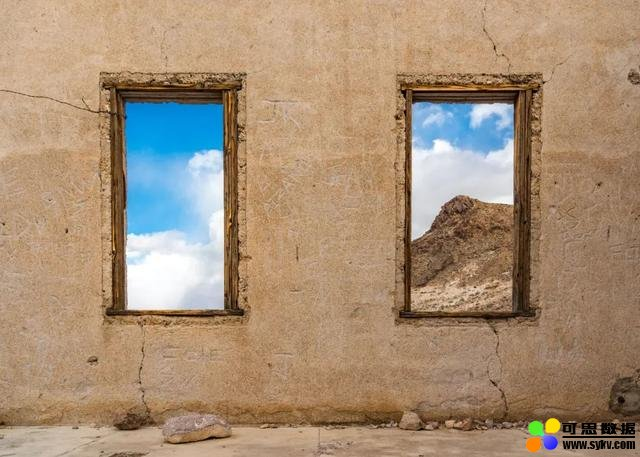1. import cv2
4.        image_in_gray_scale = cv2.cvtColor(group_of_people_image,cv2.COLOR_BGR2GRAY)
5.               faces = frontal_face_classifier.detectMultiScale(image=image_in_gray_scale,scaleFactor=1.3, minNeighbors=6
6.               for (x_axis, y_axis, weight,height) in faces:
7.            cv2.rectangle(group_of_people_image,(x_axis, y_axis), (x_axis + weight, y_axis + height), (255, 0, 0), 2)Dlib是一个拥有一些分类器的库，可以帮助我们检测人脸的某些部分，例如：眼睛、眉毛、鼻子和洋娃娃的区域。以下图为例：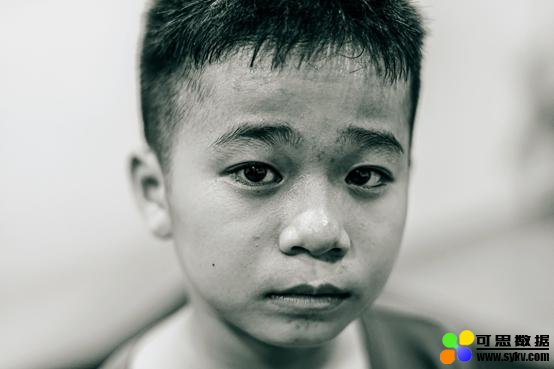1. import cv2
2.        import dlib
3.        import numpy as np
5.        initial_image_in_rgb = cv2.cvtColor(initial_image,cv2.COLOR_BGR2RGB)
6.        reference_image = initial_image_in_rgb.copy()
7.               classifier_path = dlib.shape_predictor('classifier/shape_predictor_68_face_landmarks.dat')
8.        frontal_face_detector = dlib.get_frontal_face_detector()
9.               rectangles =frontal_face_detector(initial_image,1)
10.               for k, d inenumerate(rectangles):
11.            cv2.rectangle(reference_image,(d.left(), d.top()), (d.right(), d.bottom()), (255, 255, 0), 2)
12.               landmarks = []
13.               for rectangle in rectangles:
14.            landmarks.append(np.matrix([[p.x, p.y] for p inclassifier_path(reference_image,rectangle).parts()]))
15.               for landmark in landmarks:
16.            for index, point inenumerate(landmark):
17.                point_center = (point[0, 0], point[0, 1])
18.                cv2.circle(reference_image,point_center, 3, (255, 255, 0), -1)
19.                cv2.putText(reference_image,str(index), point_center, cv2.FONT_HERSHEY_COMPLEX, 3, (255, 255, 255), 2)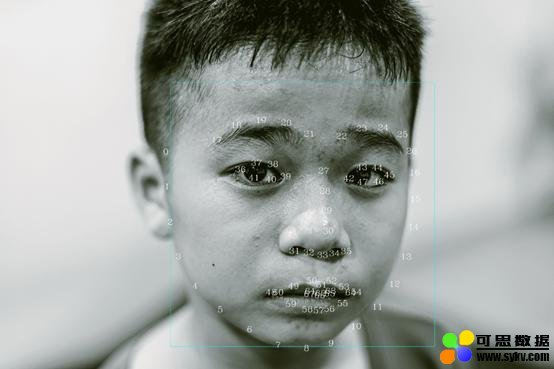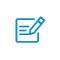扫码入群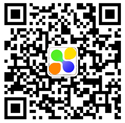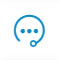咨询反馈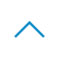返回顶部